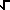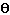# Math2.org Math Tables: Vectors(Math)

Notation: The lower case letters a-h, l-z denote scalars.Uppercase bold A-Z denote vectors. Lowercase boldi, j, k denote unit vectors. <a, b>denotes a vector with components a and b. <x1, .., xn>denotes vector with n components of which are x1, x2, x3, ..,xn. |R| denotes the magnitude of the vector R.

|<a, b>| = magnitude of vector =(a 2+ b 2)

|<x1, .., xn>| =(x12+ .. + xn2)

<a, b> + <c, d> = <a+c, b+d>

<x1, .., xn> + <y1, .., yn>= < x1+y1, .., xn+yn>

k <a, b> = <ka, kb>

k <x1, .., xn> = <k x1, .., k x2>

<a, b><c, d> = ac + bd

<x1, .., xn><y1, ..,yn> = x1 y1 + .. + xn yn>

RS= |R| |S| cos(= angle betweenthem)

RS= SR

(a R)(bS) = (ab) RS

R(S + T)= RS+ RT

RR = |R| 2

|R x S| = |R| |S| sin(= angle betweenboth vectors). Direction of R x S is perpendicularto A & B and according to the right hand rule.

``` | i j k | R x S = |
r1 r2 r3 | = / |r2
r3| |r3 r1| |r1
r2| \ | s1 s2 s3 |
\ |s2 s3| , |s3 s1|
, |s1 s2| /```

S x R = - R x S

(a R) x S = R x (a S) = a (Rx S)

R x (S + T) = R x S + Rx T

R x R = 0

If a, b, c = angles between the unit vectors i, j,k and R Then the direction cosines are set by:

cos a = (Ri) / |R|; cos b = (Rj) / |R|; cos c = (Rk) / |R|

|R x S| = Area of parallelogram with sides Rand S.

Component of R in the direction of S = |R|cos= (RS) / |S|(scalar result)

Projection of R in the direction of S = |R|cos= (RS) S/ |S| 2 (vector result)